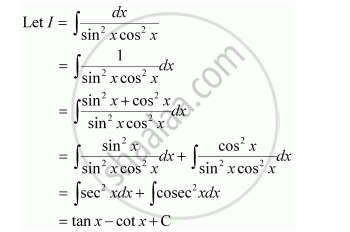Share

# Choose the Correct Answer Int (Dx)/(Sin^2 X Cos^2 X) Equals - Mathematics

#### Question

Choose the correct answer int (dx)/(sin^2 x cos^2 x) equals

(A) tan x + cot x + C

(B) tan x – cot x + C

(C) tan x cot x + C

(D) tan x – cot 2x + C

#### SolutionHence, the correct answer is B.

Is there an error in this question or solution?

#### Video TutorialsVIEW ALL 

Solution Choose the Correct Answer Int (Dx)/(Sin^2 X Cos^2 X) Equals Concept: Methods of Integration - Integration by Substitution.
S